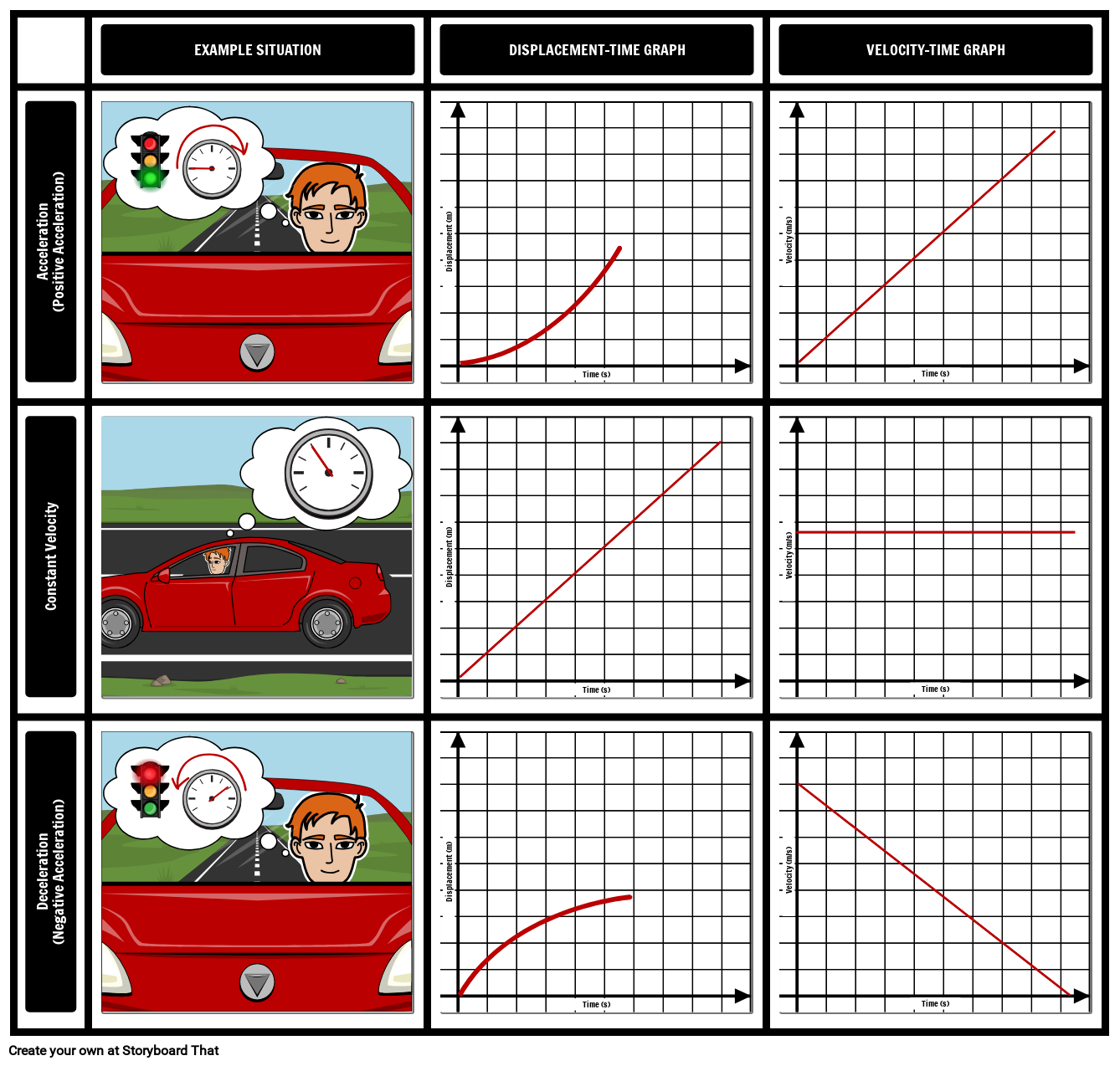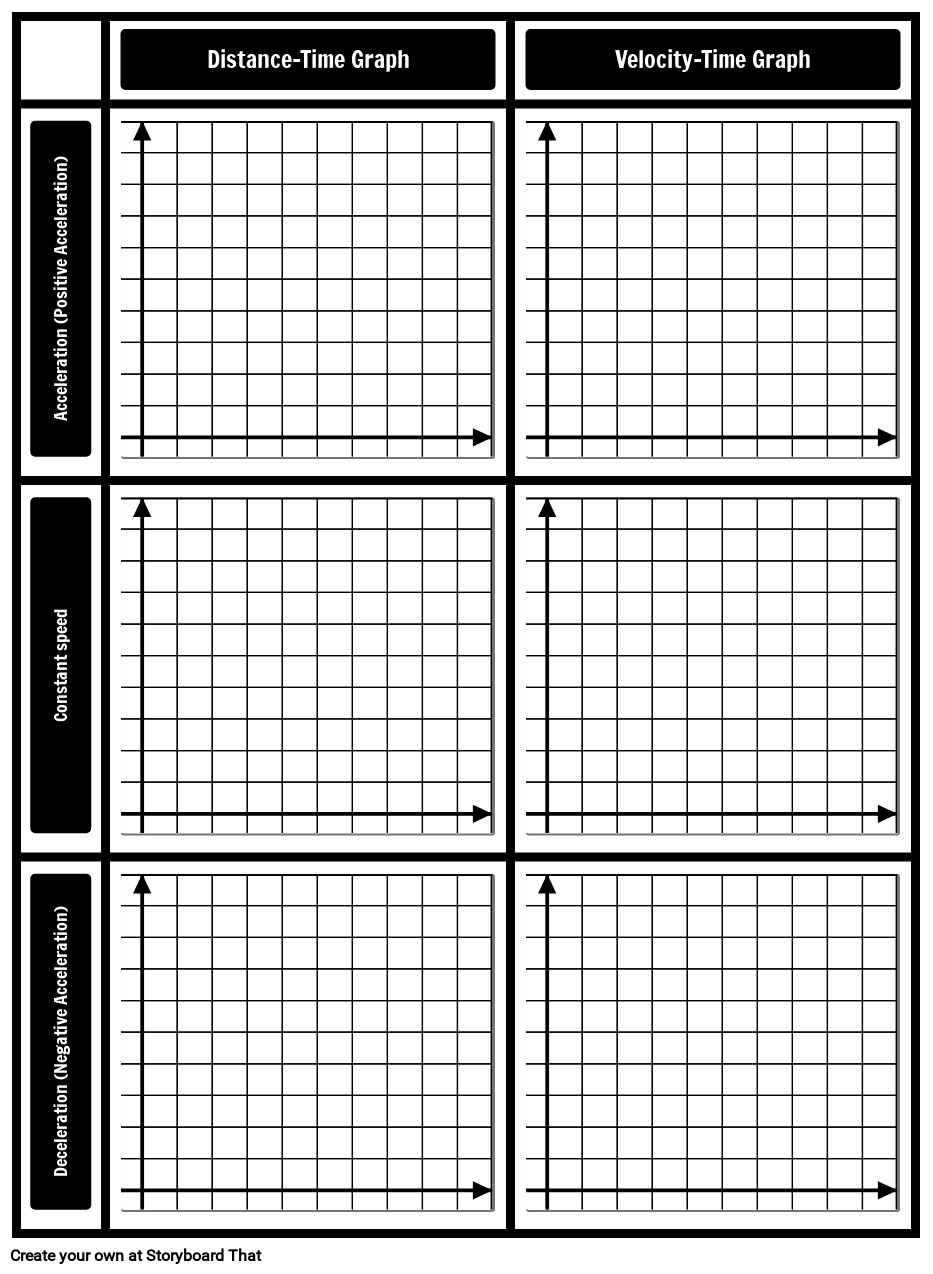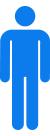Comparing Graphs## Activity Overview

Students often find it difficult to tell the difference between displacement-time graphs and velocity-time graphs. In this activity students will create a chart that illustrates how motion can be described using displacement-time graphs and velocity-time graphs. Students should be encouraged to describe the differences if they are prone to get confused even with the visual aid of the graph.

Motion TypeDisplacement-Time GraphVelocity-Time Graph
Acceleration (Positive Acceleration)A curved line where the gradient is increasingA line with a constant positive gradient
Constant VelocityA line with a constant positive gradientA horizontal line
﻿Deceleration (Negative Acceleration)﻿A curved line where the gradient is decreasingA line with a constant negative gradient

Lesson Plan Reference

Difficulty Level 4 (Difficult / Complex)

Type of Assignment Individual or Group

## Template and Class Instructions

(These instructions are completely customizable. After clicking "Use This Assignment With My Students", update the instructions on the Edit Tab of the assignment.)

#### Student Instructions

Create displacement-time and velocity-time graphs for different situations.

1. Click "Start Assignment".
2. Use a combinations of scenes, characters and props to create a visualization for the following situations: Positive acceleration, constant speed, and negative acceleration (deceleration).
3. Use lines to show what the displacement-time and velocity-time graphs look like for the following situations: Positive acceleration, constant speed, and negative acceleration (deceleration).
4. Save and submit the assignment.
Comparing Graphs Template## Rubric

(You can also create your own on Quick Rubric.)

Velocity-Time Graphs vs. Displacement-Time Graphs
Create displacement-time graphs and velocity-time graphs for a range of different given motion types.
Proficient
20 Points
Emerging
10 Points
Beginning
0 Points
Axis
Both the X and Y axis are correctly labeled.
The X or the Y axis is correctly labeled.
Neither of the axis labels are correct.
Displacement-Time Graphs
The displacement-time graphs are correct for all different motion types: acceleration (positive acceleration), constant speed, and deceleration (negative acceleration).
The displacement-time graphs are correct for two of the three different motion types: acceleration (positive acceleration), constant speed, and deceleration (negative acceleration).
The displacement-time graphs are correct for only one of the three different motion types: acceleration (positive acceleration), constant speed, and deceleration (negative acceleration).
Velocity-Time Graphs
The velocity-time graphs are correct for all different motion types: acceleration (positive acceleration), constant speed, and deceleration (negative acceleration).
The velocity-time graphs are correct for two of the three different motion types: acceleration (positive acceleration), constant speed. and deceleration (negative acceleration).
The velocity-time graphs are correct for only one of the three different motion types: acceleration (positive acceleration), constant speed, and deceleration (negative acceleration).
Visualization
Every situation has a clear and easy-to-understand visualization.
Every situation has a visualization, but some are difficult to understand.
Not every situation has a visualization.
Evidence of Effort
Work is well written and carefully thought out.
Work shows some evidence of effort.
Work shows little evidence of any effort.

## Education Pricing

This pricing structure is only available to academic institutions. Storyboard That accepts purchase orders.#### Single Teacher

As low as /month#### Department

As low as /month#### School/District

As low as /month

*(This will start a 2-Week Free Trial - No Credit Card Needed)
##### Over 15 Million Storyboards Created